Courses

# RD Sharma Solutions -Ex-10.3, Basic Geometrical Concepts, Class 6, Maths Class 6 Notes | EduRev

## RD Sharma Solutions for Class 6 Mathematics

Created by: Abhishek Kapoor

## Class 6 : RD Sharma Solutions -Ex-10.3, Basic Geometrical Concepts, Class 6, Maths Class 6 Notes | EduRev

The document RD Sharma Solutions -Ex-10.3, Basic Geometrical Concepts, Class 6, Maths Class 6 Notes | EduRev is a part of the Class 6 Course RD Sharma Solutions for Class 6 Mathematics.
All you need of Class 6 at this link: Class 6

Question 1. Draw rough diagrams to illustrate the following:
i) Open curve
ii) Closed curve

Solution :
i) Open curve –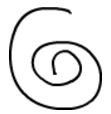ii) Closed curve –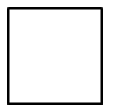Question 2. Classify the following curves as open or closed?

(i)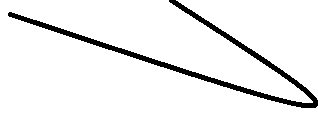Solution: Open

(ii)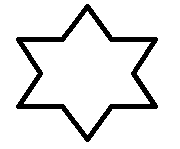Solution: Closed

(iii)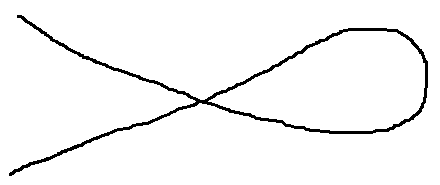Solution: Closed

(iv)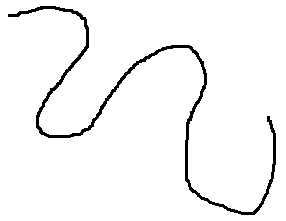Solution: Open

(v)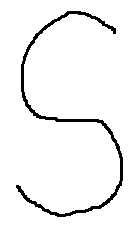Solution: Open

(vi)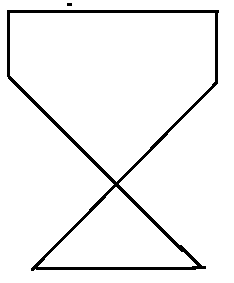Solution: Open

Question 3. Draw a polygon and shade its interior. Also draw its diagonals, if any

Solution : ABCD is a polygon and AC and BD are its two diagonals.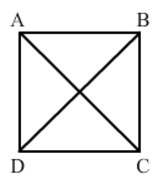Question 4. Illustrate, if possible, each one of the following with a rough diagram

i) A closed curve that is not a polygon

Solution: A circle is a simple closed curve but not a polygon. A polygon has line segments, but a circle has only curve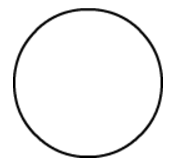ii) An open curve made up entirely of line segments

Solution: Rough diagram of an open curve made up entirely of line segments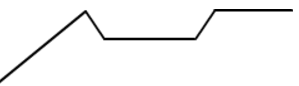iii) A polygon with two sides-

Solution: A polygon with two sides is not possible.

103 docs

,

,

,

,

,

,

,

,

,

,

,

,

,

,

,

,

,

,

,

,

,

,

,

,

,

,

,

,

,

,

;